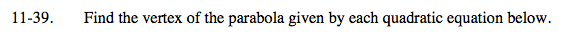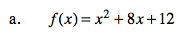Home > A2C > Chapter 11 > Lesson 11.2.1 > Problem11-39

11-39.
1. Find the vertex of the parabola given by each quadratic equation below. Homework Help ✎

1. f(x) = x2 + 8x + 12

2. g(x) = x2 − 2x + 3Convert the equation into graphing form.

(−4, −4)See part (a).